# Dual CCI Indicator36

I am trying to create a CCI indicator that allows you to plot two periods in the same indicator space, such as the 14 period and the 50 period.

I am getting these errors when I try to compile:
1)  "OnCalculate function declared with wrong type or parameters" warning

This is the code that I have so far.  Can anyone tell me what I need to change?  I am sure some of you can figure this out in 10 seconds or less.  LOL

Any help is appreciated!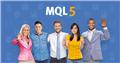Discover new MetaTrader 5 opportunities with MQL5 community and services
• www.mql5.com
Dozens of new automated trading applications appear in the MQL5 Market every day. Choose the right app among 10,000 products and forget about unnecessary routine operations of manual trading. Sell your algorithmic trading programs through the largest store of trading applications! One Click Close The script allows users to easily close...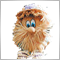Moderator
207216

I am trying to create a CCI indicator that allows you to plot two periods in the same indicator space, such as the 14 period and the 50 period.

I am getting these errors when I try to compile:
1)  "OnCalculate function declared with wrong type or parameters" warning

This is the code that I have so far.  Can anyone tell me what I need to change?  I am sure some of you can figure this out in 10 seconds or less.  LOL

Any help is appreciated!

Use button 'Code'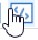36

```//+------------------------------------------------------------------+
//|                                              CCI_Two_Periods.mq5 |
//|                   Copyright 2009-2017, MetaQuotes Software Corp. |
//|                                              http://www.mql5.com |
//+------------------------------------------------------------------+
#property copyright   "2009-2017, MetaQuotes Software Corp."
#property description "Commodity Channel Index 14/50"
#include <MovingAverages.mqh>
//---
#property indicator_separate_window
#property indicator_buffers       8
#property indicator_plots         2
#property indicator_type1         DRAW_LINE
#property indicator_color1        LightSeaGreen
#property indicator_level1       -100.0
#property indicator_level2        100.0
#property indicator_level3       -200.0
#property indicator_level4        200.0
#property indicator_applied_price PRICE_TYPICAL
//--- input parameters
input int  InpCCIPeriod=14;  // Period
input int  InpCCIPeriod2=50; // Period2
//--- global variable
int        ExtCCIPeriod;
int        ExtCCIPeriod2;
//---- indicator buffer Period 1
double     ExtSPBuffer[];
double     ExtDBuffer[];
double     ExtMBuffer[];
double     ExtCCIBuffer[];
//---- indicator buffer Period 2
double     ExtSPBuffer2[];
double     ExtDBuffer2[];
double     ExtMBuffer2[];
double     ExtCCIBuffer2[];
//+------------------------------------------------------------------+
//| Custom indicator initialization function                         |
//+------------------------------------------------------------------+
void OnInit()
{
//--- check for input value of period
if(InpCCIPeriod<=0)
{
ExtCCIPeriod=14;
printf("Incorrect value for input variable InpCCIPeriod=%d. Indicator will use value=%d for calculations.",InpCCIPeriod,ExtCCIPeriod);
}
else ExtCCIPeriod=InpCCIPeriod;

if(InpCCIPeriod2<=0)
{
ExtCCIPeriod2=50;
printf("Incorrect value for input variable InpCCIPeriod2=%d. Indicator will use value=%d for calculations.",InpCCIPeriod2,ExtCCIPeriod2);
}
else ExtCCIPeriod2=InpCCIPeriod2;

//--- define buffers
SetIndexBuffer(0,ExtCCIBuffer);
SetIndexBuffer(1,ExtDBuffer,INDICATOR_CALCULATIONS);
SetIndexBuffer(2,ExtMBuffer,INDICATOR_CALCULATIONS);
SetIndexBuffer(3,ExtSPBuffer,INDICATOR_CALCULATIONS);

SetIndexBuffer(0,ExtCCIBuffer2);
SetIndexBuffer(1,ExtDBuffer2,INDICATOR_CALCULATIONS);
SetIndexBuffer(2,ExtMBuffer2,INDICATOR_CALCULATIONS);
SetIndexBuffer(3,ExtSPBuffer2,INDICATOR_CALCULATIONS);
//--- indicator name
IndicatorSetString(INDICATOR_SHORTNAME,"CCI("+string(ExtCCIPeriod)+")");
IndicatorSetString(INDICATOR_SHORTNAME,"CCI2("+string(ExtCCIPeriod2)+")");
//--- indexes draw begin settings
PlotIndexSetInteger(0,PLOT_DRAW_BEGIN,ExtCCIPeriod-1);
PlotIndexSetInteger(0,PLOT_DRAW_BEGIN,ExtCCIPeriod2-1);
//--- number of digits of indicator value
IndicatorSetInteger(INDICATOR_DIGITS,2);
//---- OnInit done
}
//+------------------------------------------------------------------+
//| Custom indicator iteration function                              |
//+------------------------------------------------------------------+
int OnCalculate(const int rates_total,
const int rates_total2,
const int prev_calculated,
const int prev_calculated2,
const int begin,
const int begin2,
const double &price[])
{
//--- variables
int    i,j,k,m;
double dTmp,dMul=0.015/ExtCCIPeriod;
double dTmp2,dMul2=0.015/ExtCCIPeriod2;
//--- start calculation
int StartCalcPosition=(ExtCCIPeriod-1)+begin;
int StartCalcPosition2=(ExtCCIPeriod2-1)+begin2;
//--- check for bars count
if(rates_total<StartCalcPosition)
return(0);
if(rates_total2<StartCalcPosition2)
return(0);
//--- correct draw begin
if(begin>0) PlotIndexSetInteger(0,PLOT_DRAW_BEGIN,StartCalcPosition+(ExtCCIPeriod-1));
if(begin2>0) PlotIndexSetInteger(0,PLOT_DRAW_BEGIN,StartCalcPosition2+(ExtCCIPeriod2-1));
//--- calculate position
int pos=prev_calculated-1;
if(pos<StartCalcPosition)
pos=StartCalcPosition;

int pos2=prev_calculated2-1;
if(pos2<StartCalcPosition2)
pos2=StartCalcPosition2;

//--- main cycle
//--- loop for first period
for(i=pos;i<rates_total && !IsStopped();i++)
{
//--- SMA on price buffer
ExtSPBuffer[i]=SimpleMA(i,ExtCCIPeriod,price);
//--- calculate D
dTmp=0.0;
for(j=0;j<ExtCCIPeriod;j++) dTmp+=MathAbs(price[i-j]-ExtSPBuffer[i]);
ExtDBuffer[i]=dTmp*dMul;
//--- calculate M
ExtMBuffer[i]=price[i]-ExtSPBuffer[i];
//--- calculate CCI
if(ExtDBuffer[i]!=0.0) ExtCCIBuffer[i]=ExtMBuffer[i]/ExtDBuffer[i];
else                   ExtCCIBuffer[i]=0.0;
}
return(rates_total);

//--- loop for second period
for(k=pos2;k<rates_total2 && !IsStopped();k++)
{
//--- SMA on price buffer
ExtSPBuffer2[k]=SimpleMA(k,ExtCCIPeriod2,price);
//--- calculate DTmp2
dTmp2=0.0;
for(m=0;m<ExtCCIPeriod2;m++) dTmp+=MathAbs(price[k-m]-ExtSPBuffer2[k]);
ExtDBuffer2[k]=dTmp2*dMul2;
//--- calculate M
ExtMBuffer2[k]=price[k]-ExtSPBuffer2[k];
//--- calculate CCI2
if(ExtDBuffer2[k]!=0.0) ExtCCIBuffer2[k]=ExtMBuffer2[k]/ExtDBuffer2[k];
else                    ExtCCIBuffer2[k]=0.0;
}
//---- OnCalculate done. Return new prev_calculated.
return(rates_total2);
}
//+------------------------------------------------------------------+```Moderator
207216

You have ten buffers:

`#property indicator_buffers       10`

```   SetIndexBuffer(0,ExtCCIPeriod,INDICATOR_DATA);
SetIndexBuffer(1,ExtCCIPeriod2,INDICATOR_DATA);
//--- indicator buffer Period 1
SetIndexBuffer(2,ExtSPBuffer,INDICATOR_CALCULATIONS)
SetIndexBuffer(3,ExtDBuffer,INDICATOR_CALCULATIONS)
SetIndexBuffer(4,ExtMBuffer,INDICATOR_CALCULATIONS)
SetIndexBuffer(5,ExtCCIBuffer,INDICATOR_CALCULATIONS)
//--- indicator buffer Period 2
SetIndexBuffer(6,ExtSPBuffer2,INDICATOR_CALCULATIONS)
SetIndexBuffer(7,ExtDBuffer2,INDICATOR_CALCULATIONS);
SetIndexBuffer(8,ExtMBuffer2,INDICATOR_CALCULATIONS)
SetIndexBuffer(9,ExtCCIBuffer2,INDICATOR_CALCULATIONS)```36

You have ten buffers:

Whoops!  Thank you for pointing that out, Vladimir.  That means that the original CCI indicator did not have this property set correctly.  ;-(  I am still learning how to code, so any pointers like this are appreciated.

I have updated my code locally, but I am still getting the same error and warning.36

I have updated my code as I compare the Stochastic indicator code (that also has two plots) to this code.  Comments are added to help identify my changes from the code above.

```//+------------------------------------------------------------------+
//|                                              CCI_Two_Periods.mq5 |
//|                   Copyright 2009-2017, MetaQuotes Software Corp. |
//|                                              http://www.mql5.com |
//+------------------------------------------------------------------+
#property copyright   "2009-2017, MetaQuotes Software Corp."
#property description "Commodity Channel Index 14/50"
#include <MovingAverages.mqh>
//---
#property indicator_separate_window
// Changed buffers from 8 to 10
#property indicator_buffers       10
#property indicator_plots         2
#property indicator_type1         DRAW_LINE
#property indicator_type2         DRAW_LINE
#property indicator_color1        White
#property indicator_color2        Blue
#property indicator_level1       -100.0
#property indicator_level2        100.0
#property indicator_level3       -200.0
#property indicator_level4        200.0
#property indicator_applied_price PRICE_TYPICAL
//--- input parameters
input int  InpCCIPeriod=14;  // Period
input int  InpCCIPeriod2=50; // Period2
//--- global variable
int        ExtCCIPeriod;
int        ExtCCIPeriod2;
//---- indicator buffer Period 1
double     ExtSPBuffer[];
double     ExtDBuffer[];
double     ExtMBuffer[];
double     ExtCCIBuffer[];
//---- indicator buffer Period 2
double     ExtSPBuffer2[];
double     ExtDBuffer2[];
double     ExtMBuffer2[];
double     ExtCCIBuffer2[];
//+------------------------------------------------------------------+
//| Custom indicator initialization function                         |
//+------------------------------------------------------------------+
void OnInit()
{
//--- check for input value of period
if(InpCCIPeriod<=0)
{
ExtCCIPeriod=14;
printf("Incorrect value for input variable InpCCIPeriod=%d. Indicator will use value=%d for calculations.",InpCCIPeriod,ExtCCIPeriod);
}
else ExtCCIPeriod=InpCCIPeriod;

if(InpCCIPeriod2<=0)
{
ExtCCIPeriod2=50;
printf("Incorrect value for input variable InpCCIPeriod2=%d. Indicator will use value=%d for calculations.",InpCCIPeriod2,ExtCCIPeriod2);
}
else ExtCCIPeriod2=InpCCIPeriod2;

//--- define buffers
SetIndexBuffer(0,ExtCCIBuffer);
SetIndexBuffer(1,ExtDBuffer,INDICATOR_CALCULATIONS);
SetIndexBuffer(2,ExtMBuffer,INDICATOR_CALCULATIONS);
SetIndexBuffer(3,ExtSPBuffer,INDICATOR_CALCULATIONS);

SetIndexBuffer(0,ExtCCIBuffer2);
SetIndexBuffer(1,ExtDBuffer2,INDICATOR_CALCULATIONS);
SetIndexBuffer(2,ExtMBuffer2,INDICATOR_CALCULATIONS);
SetIndexBuffer(3,ExtSPBuffer2,INDICATOR_CALCULATIONS);
//--- indicator name
IndicatorSetString(INDICATOR_SHORTNAME,"CCI("+string(ExtCCIPeriod)+")");
IndicatorSetString(INDICATOR_SHORTNAME,"CCI2("+string(ExtCCIPeriod2)+")");
//--- indexes draw begin settings
PlotIndexSetInteger(0,PLOT_DRAW_BEGIN,ExtCCIPeriod-1);
// Changed statement below from 0 to 1 to match Stochastic code for multiple plots
PlotIndexSetInteger(1,PLOT_DRAW_BEGIN,ExtCCIPeriod2-1);
//--- number of digits of indicator value
IndicatorSetInteger(INDICATOR_DIGITS,2);
//---- OnInit done
}
//+------------------------------------------------------------------+
//| Custom indicator iteration function                              |
//+------------------------------------------------------------------+
int OnCalculate(const int rates_total,
const int rates_total2,
const int prev_calculated,
const int prev_calculated2,
const int begin,
const int begin2,
const double &price[])
{
//--- variables
int    i,j,k,m;
double dTmp,dMul=0.015/ExtCCIPeriod;
double dTmp2,dMul2=0.015/ExtCCIPeriod2;
//--- start calculation
int StartCalcPosition=(ExtCCIPeriod-1)+begin;
int StartCalcPosition2=(ExtCCIPeriod2-1)+begin2;
//--- check for bars count
if(rates_total<StartCalcPosition)
return(0);
if(rates_total2<StartCalcPosition2)
return(0);
//--- correct draw begin
if(begin>0)  PlotIndexSetInteger(0,PLOT_DRAW_BEGIN,StartCalcPosition +(ExtCCIPeriod-1));
if(begin2>0) PlotIndexSetInteger(0,PLOT_DRAW_BEGIN,StartCalcPosition2+(ExtCCIPeriod2-1));
//--- calculate position
int pos=prev_calculated-1;
if(pos<StartCalcPosition)
pos=StartCalcPosition;

int pos2=prev_calculated2-1;
if(pos2<StartCalcPosition2)
pos2=StartCalcPosition2;

//--- main cycle
//--- loop for first period
for(i=pos;i<rates_total && !IsStopped();i++)
{
//--- SMA on price buffer
ExtSPBuffer[i]=SimpleMA(i,ExtCCIPeriod,price);
//--- calculate D
dTmp=0.0;
for(j=0;j<ExtCCIPeriod;j++) dTmp+=MathAbs(price[i-j]-ExtSPBuffer[i]);
ExtDBuffer[i]=dTmp*dMul;
//--- calculate M
ExtMBuffer[i]=price[i]-ExtSPBuffer[i];
//--- calculate CCI
if(ExtDBuffer[i]!=0.0) ExtCCIBuffer[i]=ExtMBuffer[i]/ExtDBuffer[i];
else                   ExtCCIBuffer[i]=0.0;
}
// Commented out return statement here
//    return(rates_total);

//--- loop for second period
//   for(k=pos2;k<rates_total && !IsStopped();k++)
for(k=pos2;k<rates_total2 && !IsStopped();k++)
{
//--- SMA on price buffer
ExtSPBuffer2[k]=SimpleMA(k,ExtCCIPeriod2,price);
//--- calculate DTmp2
dTmp2=0.0;
for(m=0;m<ExtCCIPeriod2;m++) dTmp+=MathAbs(price[k-m]-ExtSPBuffer2[k]);
ExtDBuffer2[k]=dTmp2*dMul2;
//--- calculate M
ExtMBuffer2[k]=price[k]-ExtSPBuffer2[k];
//--- calculate CCI2
if(ExtDBuffer2[k]!=0.0) ExtCCIBuffer2[k]=ExtMBuffer2[k]/ExtDBuffer2[k];
else                    ExtCCIBuffer2[k]=0.0;
}
//---- OnCalculate done. Return new prev_calculated.
return(rates_total);

}
//+------------------------------------------------------------------+```36

I was able to get rid of the errors by reviewing the documentation for the OnCalculate function.  The two plots do not plot correctly, though.

I get only one plot (bottom indicator in white should match the CCI 14 plot in gold) and that does not look correct when compared to standalone CCI plots for the 14 period and the 50 period.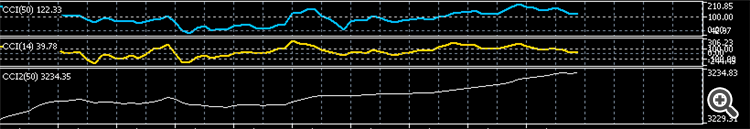This is the most recent version of the code.  Any suggestions are appreciated!

```//+------------------------------------------------------------------+
//|                                              CCI_Two_Periods.mq5 |
//|                   Copyright 2009-2017, MetaQuotes Software Corp. |
//|                                              http://www.mql5.com |
//+------------------------------------------------------------------+
#property copyright   "2009-2017, MetaQuotes Software Corp."
#property description "Commodity Channel Index 14/50"
#include <MovingAverages.mqh>
//---
#property indicator_separate_window
// Changed buffers from 8 to 10
#property indicator_buffers       10
#property indicator_plots         2
#property indicator_type1         DRAW_LINE
#property indicator_type2         DRAW_LINE
#property indicator_color1        White
#property indicator_color2        Blue
#property indicator_level1       -100.0
#property indicator_level2        100.0
#property indicator_level3       -200.0
#property indicator_level4        200.0
#property indicator_applied_price PRICE_TYPICAL
//--- input parameters
input int  InpCCIPeriod=14;  // Period
input int  InpCCIPeriod2=50; // Period2
//--- global variable
int        ExtCCIPeriod;
int        ExtCCIPeriod2;
//---- indicator buffer Period 1
double     ExtSPBuffer[];
double     ExtDBuffer[];
double     ExtMBuffer[];
double     ExtCCIBuffer[];
//---- indicator buffer Period 2
double     ExtSPBuffer2[];
double     ExtDBuffer2[];
double     ExtMBuffer2[];
double     ExtCCIBuffer2[];
//+------------------------------------------------------------------+
//| Custom indicator initialization function                         |
//+------------------------------------------------------------------+
void OnInit()
{
//--- check for input value of period
if(InpCCIPeriod<=0)
{
ExtCCIPeriod=14;
printf("Incorrect value for input variable InpCCIPeriod=%d. Indicator will use value=%d for calculations.",InpCCIPeriod,ExtCCIPeriod);
}
else ExtCCIPeriod=InpCCIPeriod;

if(InpCCIPeriod2<=0)
{
ExtCCIPeriod2=50;
printf("Incorrect value for input variable InpCCIPeriod2=%d. Indicator will use value=%d for calculations.",InpCCIPeriod2,ExtCCIPeriod2);
}
else ExtCCIPeriod2=InpCCIPeriod2;

//--- define buffers
SetIndexBuffer(0,ExtCCIBuffer);
SetIndexBuffer(1,ExtDBuffer,INDICATOR_CALCULATIONS);
SetIndexBuffer(2,ExtMBuffer,INDICATOR_CALCULATIONS);
SetIndexBuffer(3,ExtSPBuffer,INDICATOR_CALCULATIONS);

SetIndexBuffer(0,ExtCCIBuffer2);
SetIndexBuffer(1,ExtDBuffer2,INDICATOR_CALCULATIONS);
SetIndexBuffer(2,ExtMBuffer2,INDICATOR_CALCULATIONS);
SetIndexBuffer(3,ExtSPBuffer2,INDICATOR_CALCULATIONS);
//--- indicator name
IndicatorSetString(INDICATOR_SHORTNAME,"CCI("+string(ExtCCIPeriod)+")");
IndicatorSetString(INDICATOR_SHORTNAME,"CCI2("+string(ExtCCIPeriod2)+")");
//--- indexes draw begin settings
PlotIndexSetInteger(0,PLOT_DRAW_BEGIN,ExtCCIPeriod-1);
// Changed statement below from 0 to 1 to match Stochastic code for multiple plots
PlotIndexSetInteger(1,PLOT_DRAW_BEGIN,ExtCCIPeriod2-1);
//--- number of digits of indicator value
IndicatorSetInteger(INDICATOR_DIGITS,2);
//---- OnInit done
}
//+------------------------------------------------------------------+
//| Custom indicator iteration function                              |
//+------------------------------------------------------------------+
int OnCalculate(const int rates_total,
//  const int rates_total2,
const int prev_calculated,
//  const int prev_calculated2,
const int begin,
//  const int begin2,
const double &price[])
{
//--- variables
int    i,j,k,m;
double dTmp,dMul=0.015/ExtCCIPeriod;
double dTmp2,dMul2=0.015/ExtCCIPeriod2;
//--- start calculation
int StartCalcPosition=(ExtCCIPeriod-1)+begin;
//int StartCalcPosition2=(ExtCCIPeriod2-1)+begin2;
int StartCalcPosition2=(ExtCCIPeriod2-1)+begin;
//--- check for bars count
if(rates_total<StartCalcPosition)
return(0);
//   if(rates_total2<StartCalcPosition2)
//      return(0);
//--- correct draw begin
if(begin>0)  PlotIndexSetInteger(0,PLOT_DRAW_BEGIN,StartCalcPosition +(ExtCCIPeriod-1));
// if(begin2>0) PlotIndexSetInteger(0,PLOT_DRAW_BEGIN,StartCalcPosition2+(ExtCCIPeriod2-1));
if(begin>0) PlotIndexSetInteger(1,PLOT_DRAW_BEGIN,StartCalcPosition2+(ExtCCIPeriod2-1));
//--- calculate position
int pos=prev_calculated-1;
if(pos<StartCalcPosition)
pos=StartCalcPosition;

//   int pos2=prev_calculated2-1;
int pos2=prev_calculated-1;
if(pos2<StartCalcPosition2)
pos2=StartCalcPosition2;

//--- main cycle
//--- loop for first period
for(i=pos;i<rates_total && !IsStopped();i++)
{
//--- SMA on price buffer
ExtSPBuffer[i]=SimpleMA(i,ExtCCIPeriod,price);
//--- calculate D
dTmp=0.0;
for(j=0;j<ExtCCIPeriod;j++) dTmp+=MathAbs(price[i-j]-ExtSPBuffer[i]);
ExtDBuffer[i]=dTmp*dMul;
//--- calculate M
ExtMBuffer[i]=price[i]-ExtSPBuffer[i];
//--- calculate CCI
if(ExtDBuffer[i]!=0.0) ExtCCIBuffer[i]=ExtMBuffer[i]/ExtDBuffer[i];
else                   ExtCCIBuffer[i]=0.0;
}
// Commented out return statement here
//    return(rates_total);

//--- loop for second period
for(k=pos2;k<rates_total && !IsStopped();k++)
//     for(k=pos2;k<rates_total2 && !IsStopped();k++)
{
//--- SMA on price buffer
ExtSPBuffer2[k]=SimpleMA(k,ExtCCIPeriod2,price);
//--- calculate DTmp2
dTmp2=0.0;
for(m=0;m<ExtCCIPeriod2;m++) dTmp+=MathAbs(price[k-m]-ExtSPBuffer2[k]);
ExtDBuffer2[k]=dTmp2*dMul2;
//--- calculate M
ExtMBuffer2[k]=price[k]-ExtSPBuffer2[k];
//--- calculate CCI2
if(ExtDBuffer2[k]!=0.0) ExtCCIBuffer2[k]=ExtMBuffer2[k]/ExtDBuffer2[k];
else                    ExtCCIBuffer2[k]=0.0;
}
//---- OnCalculate done. Return new prev_calculated.
return(rates_total);

}
//+------------------------------------------------------------------+```Moderator
207216

Dual CCI Indicator

***

```   SetIndexBuffer(0,ExtCCIPeriod,INDICATOR_DATA);
SetIndexBuffer(1,ExtCCIPeriod2,INDICATOR_DATA);
//--- indicator buffer Period 1
SetIndexBuffer(2,ExtSPBuffer,INDICATOR_CALCULATIONS)
SetIndexBuffer(3,ExtDBuffer,INDICATOR_CALCULATIONS)
SetIndexBuffer(4,ExtMBuffer,INDICATOR_CALCULATIONS)
SetIndexBuffer(5,ExtCCIBuffer,INDICATOR_CALCULATIONS)
//--- indicator buffer Period 2
SetIndexBuffer(6,ExtSPBuffer2,INDICATOR_CALCULATIONS)
SetIndexBuffer(7,ExtDBuffer2,INDICATOR_CALCULATIONS);
SetIndexBuffer(8,ExtMBuffer2,INDICATOR_CALCULATIONS)
SetIndexBuffer(9,ExtCCIBuffer2,INDICATOR_CALCULATIONS)```36

I have updated the code with the correct buffer assignment as Vladimir suggested.  I am getting the CCI 14 period plot correctly now.  The CCI 50 plot does not display, though. I have attached a snapshot of the actual indicator and what mine is plotting now.

Any suggestions?

```//+------------------------------------------------------------------+
//|                                            CCI_Two_Periodsv2.mq5 |
//|                   Copyright 2009-2017, MetaQuotes Software Corp. |
//|                                              http://www.mql5.com |
//+------------------------------------------------------------------+
#property copyright   "2009-2017, MetaQuotes Software Corp."
#property description "Commodity Channel Index 14/50"
#include <MovingAverages.mqh>
//---
#property indicator_separate_window
// Changed buffers from 10 to 8 to get rid of error
#property indicator_buffers       8
#property indicator_plots         2
#property indicator_type1         DRAW_LINE
#property indicator_type2         DRAW_LINE
#property indicator_color1        White
#property indicator_color2        Blue
#property indicator_level1       -100.0
#property indicator_level2        100.0
#property indicator_level3       -200.0
#property indicator_level4        200.0
#property indicator_applied_price PRICE_TYPICAL
//--- input parameters
input int  InpCCIPeriod=14;  // Period
input int  InpCCIPeriod2=50; // Period2
//--- global variable
int        ExtCCIPeriod;
int        ExtCCIPeriod2;
//---- indicator buffer Period 1
double     ExtSPBuffer[];
double     ExtDBuffer[];
double     ExtMBuffer[];
double     ExtCCIBuffer[];
//---- indicator buffer Period 2
double     ExtSPBuffer2[];
double     ExtDBuffer2[];
double     ExtMBuffer2[];
double     ExtCCIBuffer2[];
//+------------------------------------------------------------------+
//| Custom indicator initialization function                         |
//+------------------------------------------------------------------+
void OnInit()
{
//--- check for input value of period
if(InpCCIPeriod<=0)
{
ExtCCIPeriod=14;
printf("Incorrect value for input variable InpCCIPeriod=%d. Indicator will use value=%d for calculations.",InpCCIPeriod,ExtCCIPeriod);
}
else ExtCCIPeriod=InpCCIPeriod;

if(InpCCIPeriod2<=0)
{
ExtCCIPeriod2=50;
printf("Incorrect value for input variable InpCCIPeriod2=%d. Indicator will use value=%d for calculations.",InpCCIPeriod2,ExtCCIPeriod2);
}
else ExtCCIPeriod2=InpCCIPeriod2;

//--- define buffers
SetIndexBuffer(0,ExtCCIBuffer);
SetIndexBuffer(1,ExtDBuffer,INDICATOR_CALCULATIONS);
SetIndexBuffer(2,ExtMBuffer,INDICATOR_CALCULATIONS);
SetIndexBuffer(3,ExtSPBuffer,INDICATOR_CALCULATIONS);

SetIndexBuffer(4,ExtCCIBuffer2);
SetIndexBuffer(5,ExtDBuffer2,INDICATOR_CALCULATIONS);
SetIndexBuffer(6,ExtMBuffer2,INDICATOR_CALCULATIONS);
SetIndexBuffer(7,ExtSPBuffer2,INDICATOR_CALCULATIONS);

//--- indicator name
IndicatorSetString(INDICATOR_SHORTNAME,"CCI("+string(ExtCCIPeriod)+","+string(ExtCCIPeriod2)+")");

//--- indexes draw begin settings
PlotIndexSetInteger(0,PLOT_DRAW_BEGIN,ExtCCIPeriod-1);
// Changed statement below from 0 to 1 to match Stochastic code for multiple plots
PlotIndexSetInteger(1,PLOT_DRAW_BEGIN,ExtCCIPeriod2-1);
//--- number of digits of indicator value
IndicatorSetInteger(INDICATOR_DIGITS,2);
//---- OnInit done
}
//+------------------------------------------------------------------+
//| Custom indicator iteration function                              |
//+------------------------------------------------------------------+
int OnCalculate(const int rates_total,
//  const int rates_total2,
const int prev_calculated,
//  const int prev_calculated2,
const int begin,
//  const int begin2,
const double &price[])
{
//--- variables
int    i,j,k,m;
double dTmp,dMul=0.015/ExtCCIPeriod;
double dTmp2,dMul2=0.015/ExtCCIPeriod2;
//--- start calculation
int StartCalcPosition=(ExtCCIPeriod-1)+begin;
//int StartCalcPosition2=(ExtCCIPeriod2-1)+begin2;
int StartCalcPosition2=(ExtCCIPeriod2-1)+begin;
//--- check for bars count
if(rates_total<StartCalcPosition)
return(0);

//--- correct draw begin
if(begin>0)  PlotIndexSetInteger(0,PLOT_DRAW_BEGIN,StartCalcPosition +(ExtCCIPeriod-1));
// Changing the first # to match the buffer #
//   if(begin>0) PlotIndexSetInteger(1,PLOT_DRAW_BEGIN,StartCalcPosition2+(ExtCCIPeriod2-1));
if(begin>0) PlotIndexSetInteger(4,PLOT_DRAW_BEGIN,StartCalcPosition2+(ExtCCIPeriod2-1));

//--- calculate position
int pos=prev_calculated-1;
if(pos<StartCalcPosition)
pos=StartCalcPosition;

//   int pos2=prev_calculated2-1;
int pos2=prev_calculated-1;
if(pos2<StartCalcPosition2)
pos2=StartCalcPosition2;

//--- main cycle
//--- loop for first period
for(i=pos;i<rates_total && !IsStopped();i++)
{
//--- SMA on price buffer
ExtSPBuffer[i]=SimpleMA(i,ExtCCIPeriod,price);
//--- calculate D
dTmp=0.0;
for(j=0;j<ExtCCIPeriod;j++) dTmp+=MathAbs(price[i-j]-ExtSPBuffer[i]);
ExtDBuffer[i]=dTmp*dMul;
//--- calculate M
ExtMBuffer[i]=price[i]-ExtSPBuffer[i];
//--- calculate CCI
if(ExtDBuffer[i]!=0.0) ExtCCIBuffer[i]=ExtMBuffer[i]/ExtDBuffer[i];
else                   ExtCCIBuffer[i]=0.0;
}
// Commented out return statement here
//    return(rates_total);

//--- loop for second period
for(k=pos2;k<rates_total && !IsStopped();k++)
//     for(k=pos2;k<rates_total2 && !IsStopped();k++)
{
//--- SMA on price buffer
ExtSPBuffer2[k]=SimpleMA(k,ExtCCIPeriod2,price);
//--- calculate DTmp2
dTmp2=0.0;
for(m=0;m<ExtCCIPeriod2;m++) dTmp2+=MathAbs(price[k-m]-ExtSPBuffer2[k]);
ExtDBuffer2[k]=dTmp2*dMul2;
//--- calculate M
ExtMBuffer2[k]=price[k]-ExtSPBuffer2[k];
//--- calculate CCI2
if(ExtDBuffer2[k]!=0.0) ExtCCIBuffer2[k]=ExtMBuffer2[k]/ExtDBuffer2[k];
else                    ExtCCIBuffer2[k]=0.0;
}
//---- OnCalculate done. Return new prev_calculated.
return(rates_total);

}```
Files:
CCI_plots.png 14 kbModerator
207216

You messed up the buffers

```//--- global variable
int        ExtCCIPeriod;
int        ExtCCIPeriod2;
//---- indicator buffer Period 1
double     ExtSPBuffer[];
double     ExtDBuffer[];
double     ExtMBuffer[];
double     ExtCCIBuffer[];
//---- indicator buffer Period 2
double     ExtSPBuffer2[];
double     ExtDBuffer2[];
double     ExtMBuffer2[];
double     ExtCCIBuffer2[];
//+------------------------------------------------------------------+
//| Custom indicator initialization function                         |
//+------------------------------------------------------------------+
void OnInit()
{
//--- check for input value of period
if(InpCCIPeriod<=0)
{
ExtCCIPeriod=14;
printf("Incorrect value for input variable InpCCIPeriod=%d. Indicator will use value=%d for calculations.",InpCCIPeriod,ExtCCIPeriod);
}
else ExtCCIPeriod=InpCCIPeriod;

if(InpCCIPeriod2<=0)
{
ExtCCIPeriod2=50;
printf("Incorrect value for input variable InpCCIPeriod2=%d. Indicator will use value=%d for calculations.",InpCCIPeriod2,ExtCCIPeriod2);
}
else ExtCCIPeriod2=InpCCIPeriod2;

//--- define buffers
SetIndexBuffer(0,ExtCCIBuffer);
SetIndexBuffer(1,ExtDBuffer,INDICATOR_CALCULATIONS);
SetIndexBuffer(2,ExtMBuffer,INDICATOR_CALCULATIONS);
SetIndexBuffer(3,ExtSPBuffer,INDICATOR_CALCULATIONS);

SetIndexBuffer(4,ExtCCIBuffer2);
SetIndexBuffer(5,ExtDBuffer2,INDICATOR_CALCULATIONS);
SetIndexBuffer(6,ExtMBuffer2,INDICATOR_CALCULATIONS);
SetIndexBuffer(7,ExtSPBuffer2,INDICATOR_CALCULATIONS);```Moderator
207216

I created a step by step instruction: CCI Dual: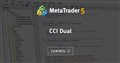CCI Dual
• www.mql5.com
Отобразить в одном подокне два индикатора iCCI ( Commodity Channel Index, CCI) с разными периодами усреднения. На примере этого индикатора я покажу, как использовать MQL Wizard и как использовать готовые решения из справки iCCI. Итак, по шагам: Даём имя индикатору Добавляем входной параметр (потом его заменим) Жмём кнопку ' Next ' Отмечаем, что...